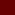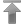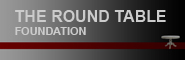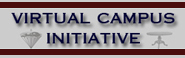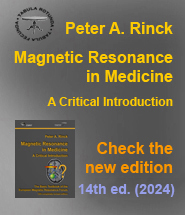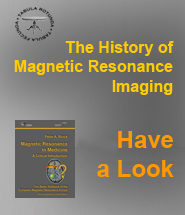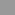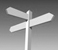Contenido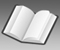Datos# Glosario

 a b c d e f g h i j k l m n o p q r s t u v w x y z

### B

B0: a conventional symbol for the constant magnetic (induction) field in an NMR sys­tem. (Although historically used, H0 (units of magnetic field strength, ampere/meter) should be distinguished from the more appropriate B0 (units of magnetic induction, Tes­la).

B1: a conventional symbol for the radiofrequency magnetic induction field used in an NMR system (another symbol historically used is H1. It is useful to consider it as com­po­sed of two oppositely rotating vectors, usually in a plane transverse to B0. At the Larmor frequency, the vector rotating in the same direction as the precessing spins will interact strongly with the spins.

Binomial pulses: a sequence of two or more pulses used to suppress the water signal in localized proton spectroscopy.

Bit: the smallest unit of information in the storage on a computer. Eight bits are grouped together to form one byte.

Bloch equations: phenomenological 'classical' equations of motion for the mac­ro­sco­pic magnetization vector. They include the effects of precession about the magnetic field (static and RF ) and the T1 and T2 relaxation times.

Boltzmann distribution: if a system of particles, which are able to exchange energy via collisions, is in thermal equilibrium, then the relative number of particles, N1 and N2, in two particular energy states with

N1 / N2 = exp - (E1 - E2) / k × T

where k is Boltzmann's constant and T is absolute temperature. For example, in NMR of protons at room temperature in a magnetic field of 0.25 Tesla, the difference in numbers of spins aligned with the magnetic field and against the field is about one part in a mil­li­on; the small excess of nuclei in the lower energy state is the basis of the net mag­ne­ti­za­tion and the resonance phenomenon.

Bulk susceptibility (agent) → Superparamagnetic.

Byte: eight bits.

Missing terms? Send us an e-mail. We'll add them ...